# Methods and formulas for Gage Linearity

Select the method or formula of your choice.

## Linearity

Linearity assesses the difference in average bias through the expected operating range of the measurement system. Linearity indicates whether the gage has the same accuracy for all sizes of objects being measured.

### Formula

Linearity = | Slope | * Process Variation

## %Linearity

%Linearity is linearity expressed as a percentage of the overall process variation.

### Formula

%Linearity = (Linearity / Process Variation) * 100

## p-value for Gage Linearity

Use the p-values for constant and slope to test whether the slope is equal to 0 and the constant is equal to 0.

The p-value is defined as the area under the sampling distribution to the right of the + |test statistic| and the area under the sampling distribution to the left of the - |test statistic|. Minitab use the t-distribution with γ df and the t-statistic to calculate the p-value.

If the p-value is greater than the α-value, you fail to reject the null hypothesis, as follows:
• For the constant, if the p-value is greater than the α-value, you fail to reject the null hypothesis and conclude that the bias for all reference values is equal to 0.
• For the slope, if the p-value is greater than the α-value, you fail to reject the null hypothesis and conclude that the measurement system has the same bias for all reference values (linearity is not present).
If the p-value is less than the α-value, you reject the null hypothesis, as follows:
• For the constant, if the p-value is less than the α-value, you reject the null hypothesis and conclude that the bias for all reference values is not equal to 0.
• For the slope, if the p-value is less than the α-value, you reject the null hypothesis and conclude that the measurement system does not have the same bias for all reference values (linearity is present).

## R-Sq

R-Sq (R2) is the coefficient of determination, which is used to check whether a fitted line models the data well. R-Sq (R2) represents the proportion of variation in the bias that is explained by the linear relationship between the biases and the reference values.

### Formula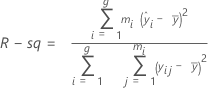### Notation

TermDescription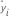ith fitted response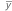mean bias
yijbias of the jth measurement of the ith part
gnumber of parts
minumber of replicates of the ith part

## S

S is an estimate of σ, the standard deviation around the regression line.

### Formula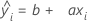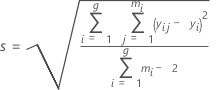### Notation

TermDescription
xireference value for part i
yijbias of the jth measurement of the ith part
gnumber of parts
minumber of replicates of the ith part

## Slope, Constant

The regression line of the Bias versus Reference value plot takes the form:

### Formulas

The slope of the regression line (a):The intercept of the regression line (b):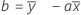### Notation

TermDescription
aslope
bintercept
xireference value for part i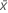average reference value across all parts
yijbias of the jth measurement of the ith part
gnumber of parts
minumber of replicates of the ith partoverall bias average
By using this site you agree to the use of cookies for analytics and personalized content.  Read our policy